# Pragmatic AI: Integrating Machine Learning with Drools

As a business analyst or business rules developer, you can integrate Machine Learning (ML) with Drools by using PMML files with Decision Model and Notation (DMN) models.

You can also integrate other Drools knowledge assets such as DRL with external Deep Learning (ML/DL) libraries. This section of the documentation is dedicated to highlight one pragmatic example, using DMN and PMML.

## Introduction to Pragmatic AI

When you think about Artificial Intelligence (AI), Machine Learning and big data might come to mind. But machine learning is only part of the picture. Artificial intelligence includes the following technologies:

• Robotics: The integration of technology, science, and engineering that produces machines that can perform physical tasks that are performed by humans

• Machine learning: The ability of a collection of algorithms to learn or improve when exposed to data without being explicitly programmed to do so

• Natural language processing: A subset of machine learning that processes human speech

• Mathematical optimization: The use of conditions and constraints to find the optimal solution to a problem

• Digital decisioning: The use of defined criteria, conditions, and a series of machine and human tasks to make decisionsWhile science fiction is filled with what is referred to as artificial general intelligence (AGI), machines that perform better than people and cannot be distinguished from them and learn and evolve without human intervention or control, AGI is decades away. Meanwhile, we have pragmatic AI which is much less frightening and much more useful to us today. Pragmatic AI is a collection of AI technologies that, when combined, provide solutions to problems such as predicting customer behavior, providing automated customer service, and helping customers make purchasing decisions.

Leading industry analysts report that previously organizations have struggled with AI technologies because they invested in the potential of AI rather than the reality of what AI can deliver today. AI projects were not productive and as a result investment in AI slowed and budgets for AI projects were reduced. This disillusionment with AI is often referred to as an AI winter. AI has experienced several cycles of AI winters followed by AI springs and we are now decidedly in an AI spring. Organizations are seeing the practical reality of what AI can deliver. Being pragmatic means being practical and realistic. A pragmatic approach to AI considers AI technologies that are available today, combines them where useful, and adds human intervention when needed to create solutions to real-world problems.

Pragmatic AI solution example

One application of pragmatic AI is in customer support. A customer files a support ticket that reports a problem, for example, a login error. A machine learning algorithm is applied to the ticket to match the ticket content with existing solutions, based on keywords or natural language processing (NLP). The keywords might appear in many solutions, some relevant and some not as relevant. You can use digital decisioning to determine which solutions to present to the customer. However, sometimes none of the solutions proposed by the algorithm are appropriate to propose to the customer. This can be because all solutions have a low confidence score or multiple solutions have a high confidence score. In cases where an appropriate solution cannot be found, the digital decisioning can involve the human support team. To find the best support person based on availability and expertise, mathematical optimization selects the best assignee for the support ticket by considering employee rostering constraints.

As this example shows, you can combine machine learning to extract information from data analysis and digital decisioning to model human knowledge and experience. You can then apply mathematical optimization to schedule human assistance. This is a pattern that you can apply to other situations, for example, a credit card dispute and credit card fraud detection.These technologies use four industry standards:

• Case Management Model and Notation (CMMN)

CMMN is used to model work methods that include various activities that might be performed in an unpredictable order depending on circumstances. CMMN models are event centered. CMMN overcomes limitations of what can be modeled with BPMN2 by supporting less structured work tasks and tasks driven by humans. By combining BPMN and CMMN you can create much more powerful models.

• Business Process Model and Notation (BPMN2)

The BPMN2 specification is an Object Management Group (OMG) specification that defines standards for graphically representing a business process, defines execution semantics for the elements, and provides process definitions in XML format. BPMN2 can model computer and human tasks.

• Decision Model and Notation (DMN)

Decision Model and Notation (DMN) is a standard established by the OMG for describing and modeling operational decisions. DMN defines an XML schema that enables DMN models to be shared between DMN-compliant platforms and across organizations so that business analysts and business rules developers can collaborate in designing and implementing DMN decision services. The DMN standard is similar to and can be used together with the Business Process Model and Notation (BPMN) standard for designing and modeling business processes.

• Predictive Model Markup Language (PMML)

PMML is the language used to represent predictive models, mathematical models that use statistical techniques to uncover, or learn, patterns in large volumes of data. Predictive models use the patterns that they learn to predict the existence of patterns in new data. With PMML, you can share predictive models between applications. This data is exported as a PMML file that can be consumed by a DMN model. As a machine learning framework continues to train the model, the updated data can be saved to the existing PMML file. This means that you can use predictive models created by any application that can save the model as a PMML file. Therefore, DMN and PMML integrate well.

Putting it all togetherThis illustration shows how predictive decision automation works.

1. Business data enters the system, for example, data from a loan application.

2. A decision model that is integrated with a predictive model decides whether or not to approve the loan or whether additional tasks are required.

3. A business action results, for example, a rejection letter or loan offer is sent to the customer.

The next section demonstrates how predictive decision automation works with Drools.

## Credit card fraud dispute use case example

The financial industry uses pragmatic AI for decisioning in several areas. One area is credit card charge disputes. When a customer identifies an incorrect or unrecognized charge on a credit card bill, the customer can dispute the charge. Human intervention in credit card fraud detection is required in some cases but the majority of reported credit card fraud can be completely or partially resolved with pragmatic AI.

This use case example is about the fictitious Fortress Bank, the bank’s customer Joe, and the business process management (BPM) developer Michelle. First we will look at how the bank originally used AI through Drools digital decisioning and then we will see how Michelle enhanced the decision model with a Predictive Model Markup Language (PMML) model created from machine learning.

Machine learning models such as Tensorflow™ and R™ produce predictive models. You can save these predictive models in an open standard such as PMML so that you can use the model with Drools or other products that support the PMML standard.

Drools and OpenShift

Fortress Bank uses Drools on OpenShift to develop and run the Fortress Bank decision services. OpenShift is a cloud platform for developing and running containerized applications, on top of Kubernetes. It is designed to enable applications and the data centers that support them to expand from just a few machines and applications to thousands of machines that serve millions of clients. You can use Drools as a platform for creating cloud-native decision services, business automation applications and microservices. Drools, in this context, enables enterprise business and IT users to document, simulate, manage, automate, and monitor business processes and decisions.

Credit card dispute digital decisioning with Drools

Joe is a Fortress Bank customer. Each month, he logs in to the Fortress Bank website to review all of the charges on his bill before he pays it. This month, Joe sees a transaction that he recognizes but the amount is incorrect. The vendor has charged him \$44.50 instead of \$4.50. Joe selects the row that contains the incorrect item and clicks Dispute.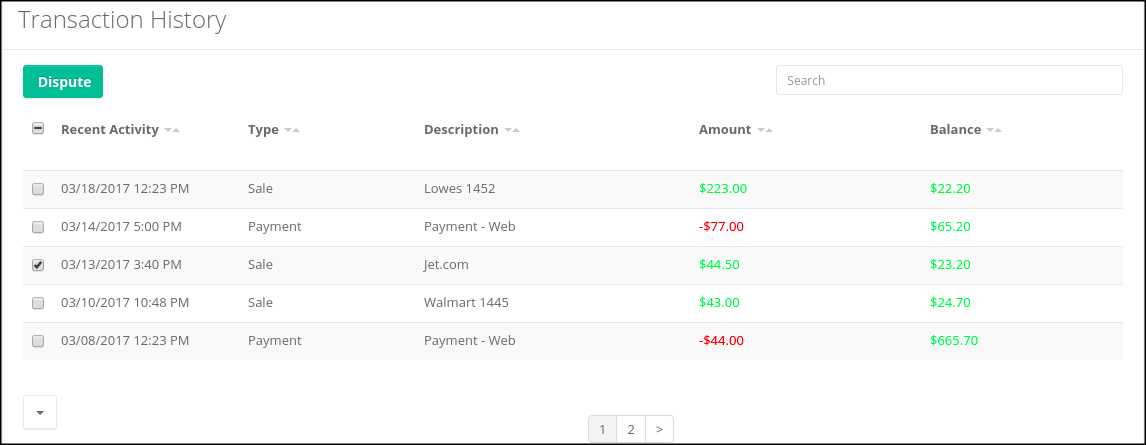This action initiates a series of questions about the dispute:

1. Why are you disputing these transactions?

2. Has your card been with you the entire time?

After Joe answers the questions, the website provides Joe with an incident number, `FR-00000004`.

Fortress Bank must now decide whether to reimburse the disputed amount without human investigation or whether to investigate the claim manually. Manual investigation requires more resources so automatic processing of the disputed amount costs the bank less in terms of human resources. However, if the bank automatically accepts all disputed amounts the cost will ultimately be greater to the bank because of money paid for fraudulent claims. Someone or something must make the decision whether or not to investigate.

Credit Card Dispute project

To help with this decisioning, Fortress Bank created the CreditCardDisputeCase project that contains the fraudDispute business process that models the dispute process.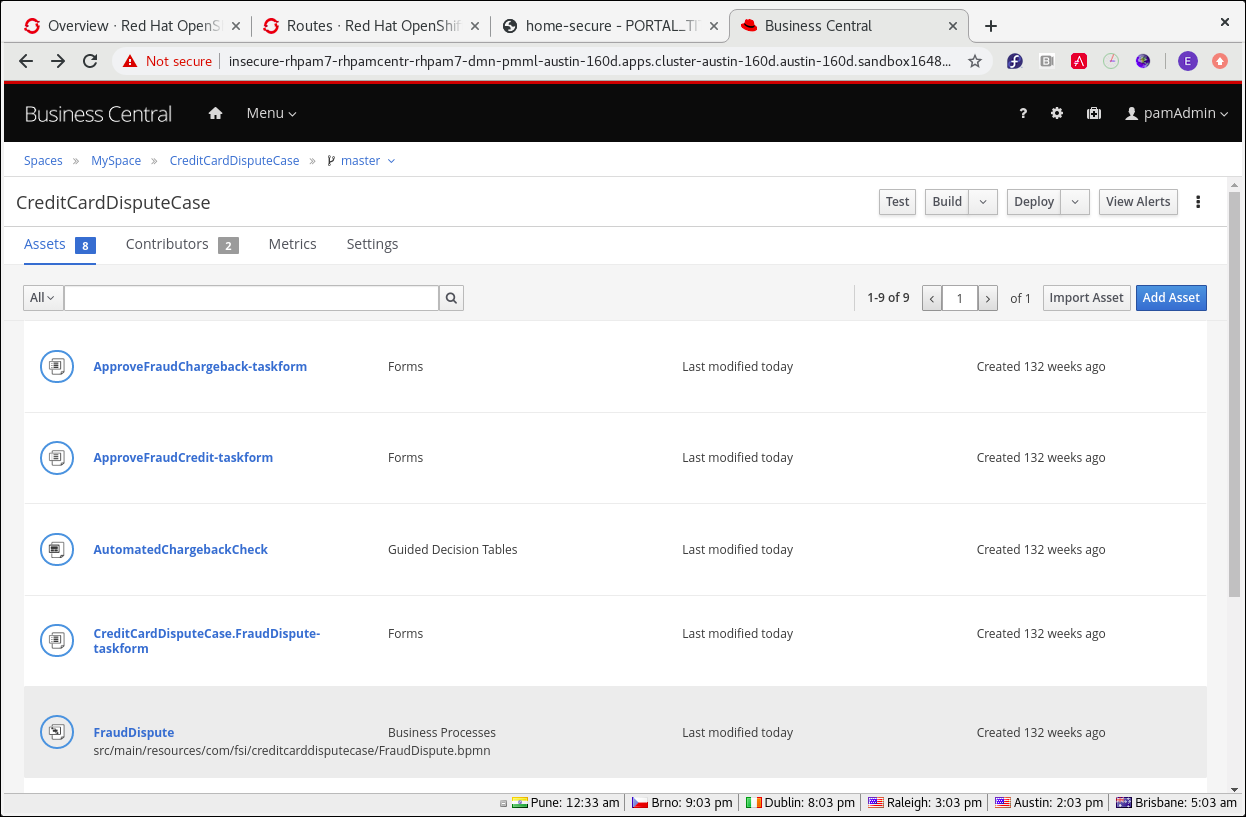Process variables

When Joe reported the dispute, an instance of the fraudDispute process was created with case ID `FR-00000004`. The Process Variables tab contains several variables and values specific to Joe’s account, including `CaseID`, `caseFile_cardholderRiskRating` (the credit card holder risk rating), and `caseFile_disputeRiskRating` (the risk rating of this dispute):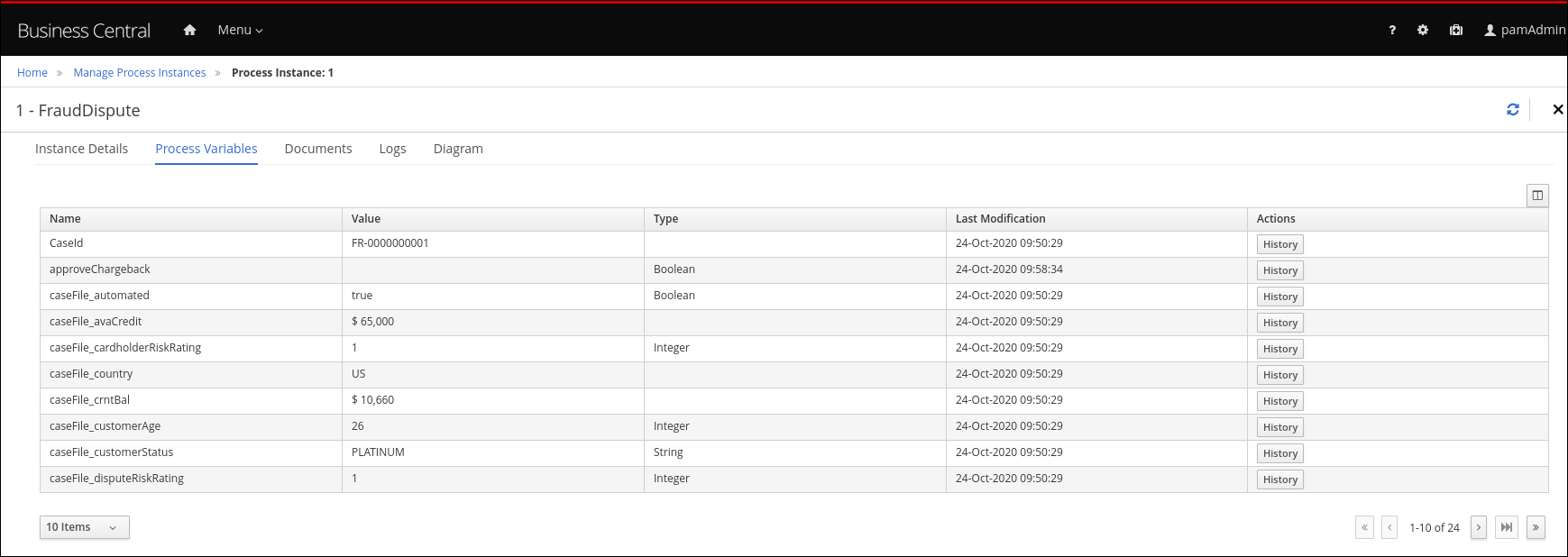The table also has the `casefile_automated` variable with the value `true`. This indicates that the dispute met the criteria to be automatically processed.

Process diagram

The Diagram tab contains the BPMN diagram that shows the decision paths that the bank uses when deciding whether to process a dispute automatically or manually: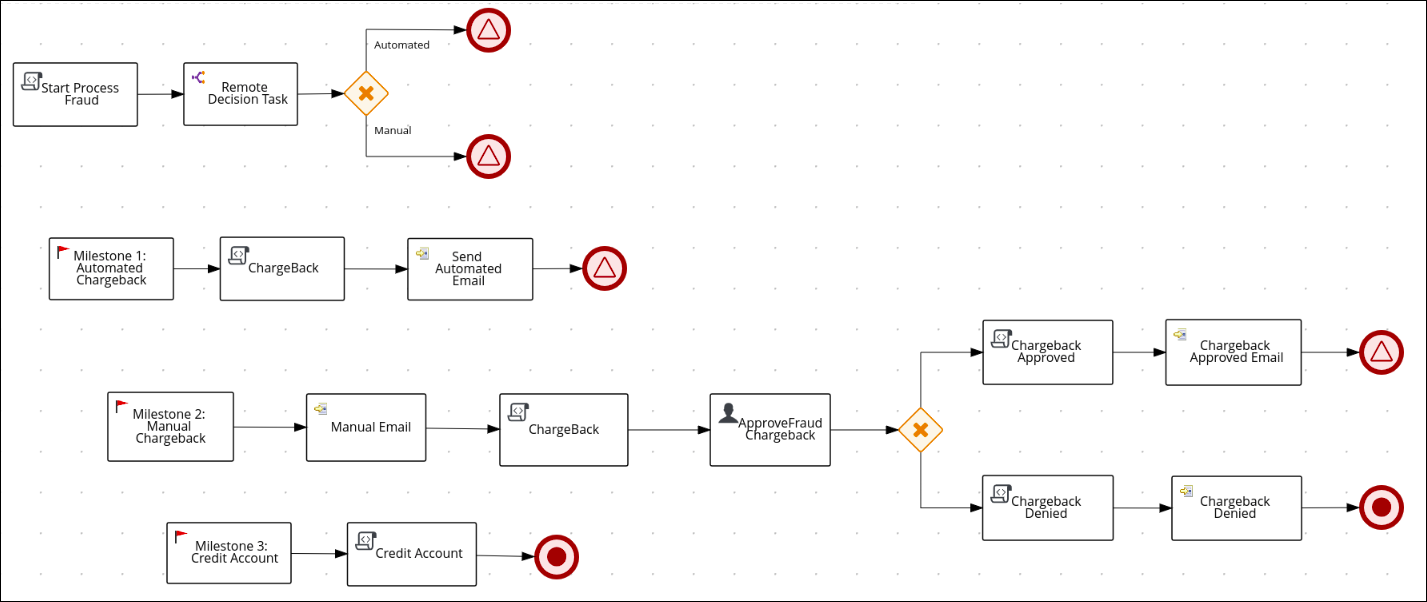The Decision Task task contains the rules that determine whether the dispute will be automatically charged back to Joe’s account (Milestone 1) or whether more investigation is required (Milestone 2) based on the values of the `caseFile_cardholderRiskRating` and the `caseFile_disputeRiskRating` variables. If Joe’s dispute matches the criteria for automatic approval, Milestone 1 is followed and the disputed amount is charged back to his account. This sub-process is very lean and efficient. If Joe’s dispute requires manual evaluation, the sub-process in Milestone 2 is started, which will require some human actor involvement and will take more resources to process.

In this case, the Decision Task task decided to process Joe’s dispute automatically so it followed Milestone 1: Automatic Chargeback.

DMN model

The following simplified DMN model shows the decision process that is part of the fraudDispute Decision Task task: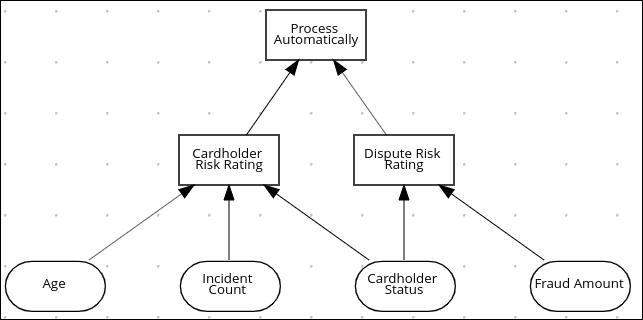The input variables are Age (cardholder age), Incident Count (the number of previous disputes for this cardholder), Cardholder Status (standard, silver, gold, platinum), and Fraud Amount.

Drools uses input variables in decision tables to support digital decisioning. The decision tables are created by human business analysts. The analyst creates a draft business requirement analysis document or spreadsheet that stakeholders review and approve. The project designer then uses the KIE DMN Editor to transfer the data in the analysis document to the DMN model. The Fortress Bank Credit Card Dispute process has two decision tables, the Cardholder Risk Rating table and the Dispute Risk Rating table. The Cardholder Risk Rating table contains three input variables: Incident Count, Cardholder Status, and Age. The Dispute Risk Rating table contains the Cardholder Status input variable. The table calculates the risk of the dispute based on the cardholder status and the amount of the dispute.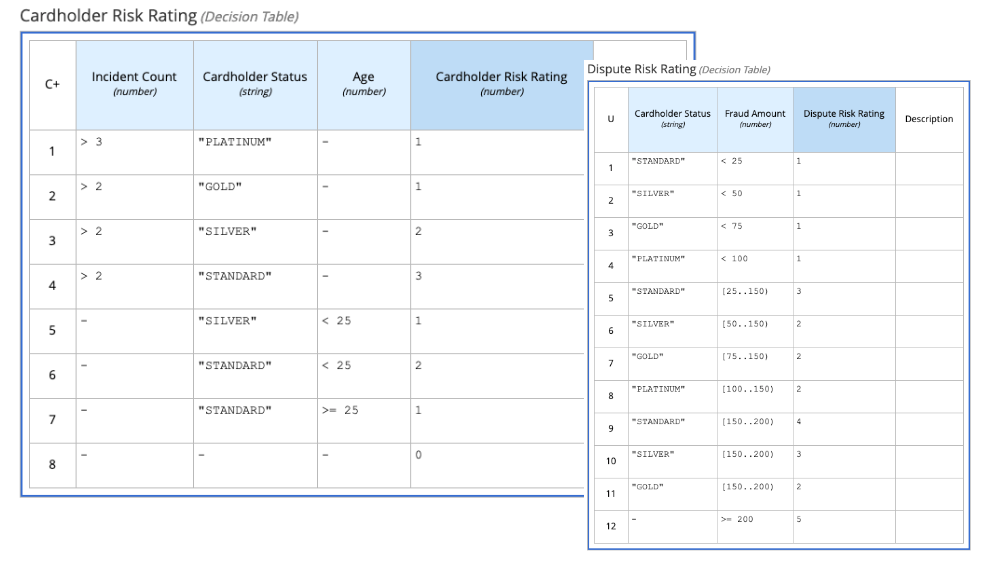• Cardholder Risk Rating

Joe is a Silver cardholder over the age of 25. He has had more than two previous disputes, so he has a risk rating of 2. If Joe had no previous disputes, he would have a risk rating of 0.

• Dispute Risk Rating

Because Joe is a Silver cardholder and the disputed amount is \$40, Joe has a rating of 1 on the Dispute Risk Rating table. If the disputed amount was \$140, Joe would have a risk rating of 2.

The following formula, which is implemented as part of the Process Automatically final decision in the DMN model, uses the scores from the two decision tables to determine whether to automatically charge back the disputed amount (Milestone 1) or whether more investigation is needed (Milestone 2).

``(Cardholder Risk Rating + Dispute Risk Rating) < 5``

If Joe’s overall risk score is less than five, his disputed amount is charged back automatically (Milestone 1). If the overall score is 5 or greater, then his dispute is processed manually (Milestone 2).

Adding machine learning to Drools digital decisioning

Because Fortress Bank has historical data about its customers, including previous transaction and dispute history, the bank can use that data with machine learning to create predictive models that can be used in the DMN model decision tasks. This results in a more accurate assessment of the risk compared to the decision tables created by business analysts.

Fortress Bank has two sets of PMML files that contain models which more accurately assess risk predictions. One set is based on the linear regression algorithm and the other is based on the random forests algorithm.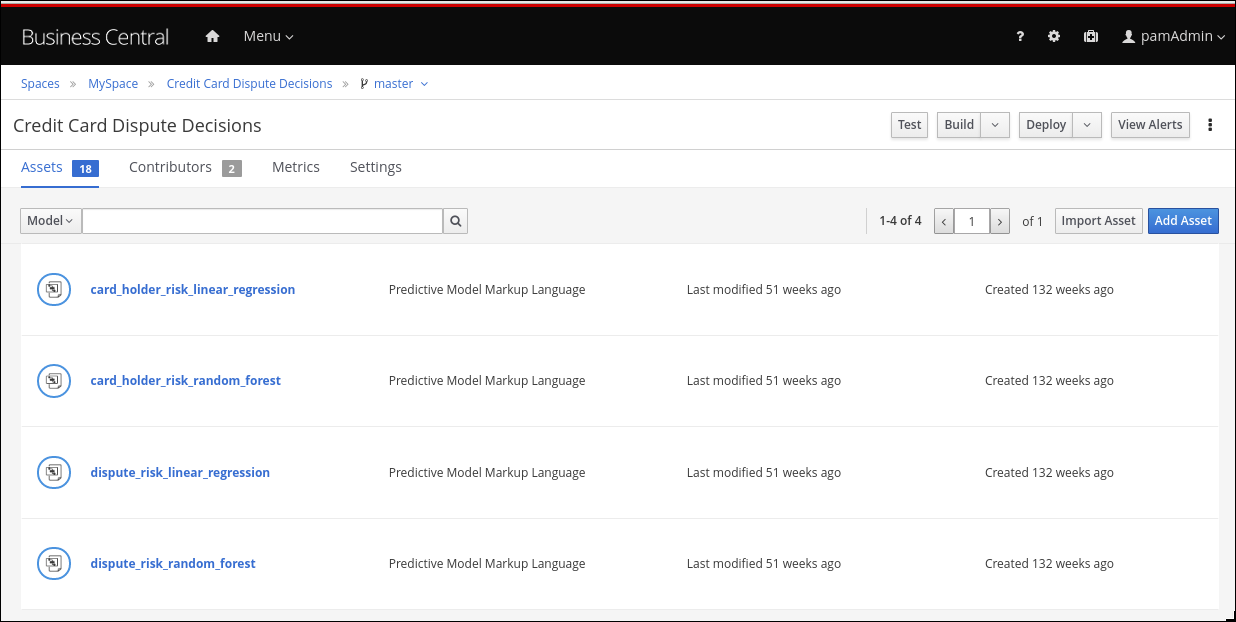Linear regression is one of the most widely used algorithms in both statistics and machine learning. It uses a linear equation that combines a set of numeric input and output values. Random forests use many decision trees as inputs to create prediction models.

Michelle imports the dispute_risk_linear_regression PMML file into her project. She adds the Cardholder Risk Model business model knowledge node to the DMN model and associates the dispute_risk_linear_regression PMML file with the node. Drools analyzes the PMML file and adds input parameters to the node. Michelle associates the Cardholder Risk Model node with the Dispute Risk Rating.

Michelle then adds the credit_card_holder_risk_linear_regression PMML model to the projects, creates the Dispute Risk Model mode DMN file, creates and associates the credit_card_holder_risk_linear_regression PMML file with the node. Drools analyzes the PMML file and adds input parameters to the node.

The following image is Michelle’s completed DMN model, which replaces analytical decision tables with the predictive models from the PMML files: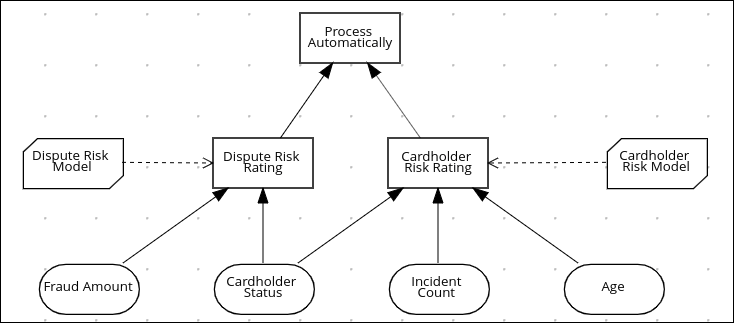Michelle now returns to the fraudDispute BPMN model and updates the model with the PMML files that she added. She then redeploys the project.

Increased score precision

In this new scenario where Michelle has redeployed the Fortress Bank project with PMML models, we can see what happens when Joe logs in to his Fortress Bank account and reports the same transaction as incorrect. At this point, Michelle navigates to the Process Instances window and she sees Joe’s new dispute instance. In the Process Variables tab, Michelle reviews the values of cardHolderRiskRating and the disputeRiskRating. They have changed because the model is now using the PMML files. This provides a much more precise prediction of risk by making use of machine learning models based on historical data. At the same time, the policy of the bank is still enforced by the DMN decision model: the risk predictor is below a specified threshold which allows for this dispute to be processed automatically.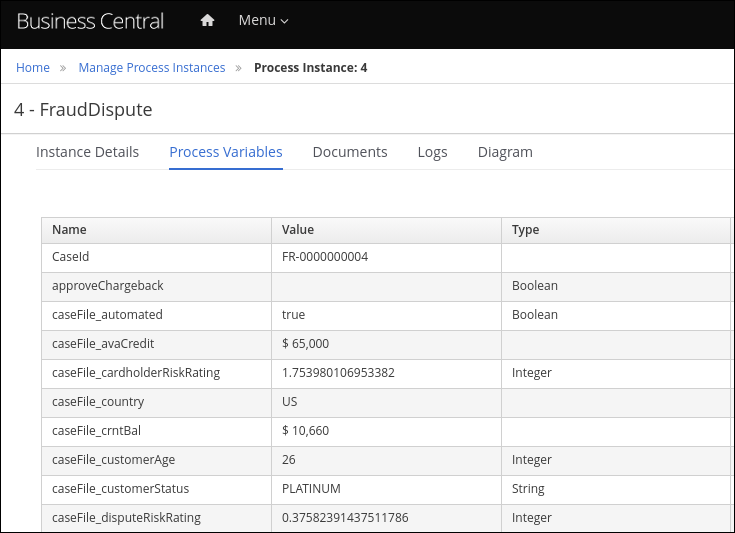Monitoring

Finally, Fortress Bank uses Prometheus to gather metrics about credit card disputes and Grafana to visualize those metrics in real time. The upper section of the monitor shows the business metrics key performance indicators (KPIs) and the lower section shows the operational metrics KPIs.### Using a PMML model with a DMN model to resolve credit card transaction disputes

This example shows you how to use Drools to create a DMN model that uses a PMML model to resolve credit card transaction disputes. When a customer disputes a credit card transaction, the system decides whether or not to process the transaction automatically.

Prerequisites
• A PMML evaluation library such as the `kie-pmml-trusty` offered as part of Drools

• Alternatively, Drools may be integrated with third-party PMML libraries such as JPMML, requiring:

• `kie-dmn-jpmml-7.59.0.Final.jar`

The group ID, artifact ID, and version (GAV) identifier of this file is `org.kie:kie-dmn-jpmml:7.59.0.Final`. For more information, see [dmn-included-models-pmml-proc_dmn-models].

• JPMML Evaluator 1.5.1 JAR file

• JPMML Evaluator Extensions 1.5.1 JAR file

These files are required to enable JPMML evaluation.

 We provide these additional plug-in modules to support the integration with the Java Evaluator API for PMML (JPMML) for PMML execution in Drools. However, we do not support the JPMML libraries directly. If you include JPMML libraries in your Drools distribution, see the Openscoring.io licensing terms for JPMML.
Procedure
1. Create the `dtree_risk_predictor.pmml` file with the contents of the XML example in Credit card transaction dispute exercise PMML file.

2. Add the `dtree_risk_predictor.pmml` file into the `com` package in your KJAR project.

3. Create the Dispute Transaction Check DMN model in com package in your KJAR project.

4. Using the KIE DMN Editor, create the tTransaction custom data type: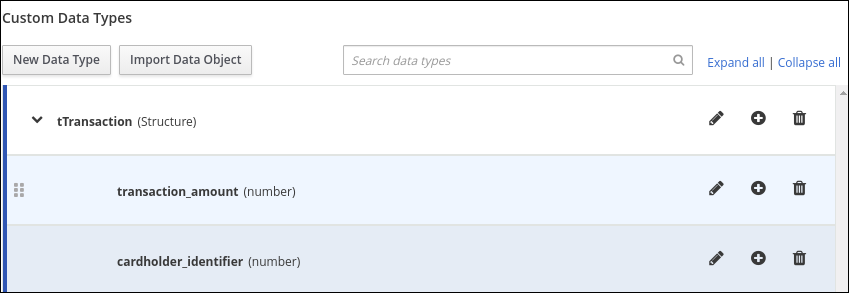1. Click the Data Types tab.

2. Click Add a custom Data Type.

3. In the Name box, enter `tTransaction`.

4. Select Structure from the Type menu.

5. To create the data type, click the check mark.

The tTransaction custom data type appears with one variable row.

6. In the Name field of the variable row, enter `transaction_amount`, select Number from the Type menu, and then click the check mark.

7. To add a new variable row, click the plus symbol on the `transaction_amount` row. A new row appears.

8. In the Name field, enter `cardholder_identifier`, select Number from the Type menu, and then click the check mark.

5. Add the Risk Predictor `dtree_risk_predictor.pmml` model: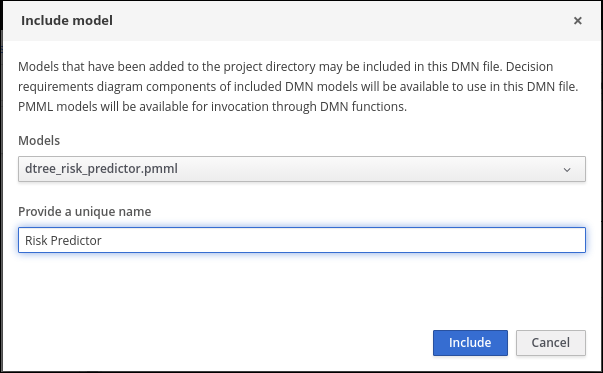1. In the Included Models window of the DMN editor, click Include Model.

2. In the Include Model dialog, select `dtree_risk_predictor.pmml` from the Models menu.

3. Enter `Risk Predictor` in the Provide a unique name box and click OK.

6. Create the Risk Predictor Business Knowledge Model (BKM) node with the Risk Predictor and DecisionTreeClassifier model: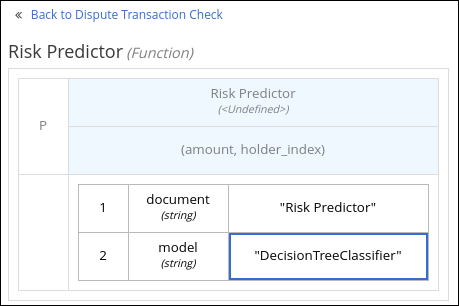1. In the Model window of the DMN editor, drag a BKM node to the DMN editor palette.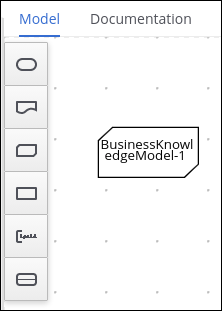2. Rename the node Risk Predictor.

3. Click the edit icon located below the trash can icon on the left side of the node.4. Click F in the Risk Predictor box and select PMML from the Select Function Kind menu. The F changes to P.

5. Double-click the First select PMML document box and select Risk Predictor.

6. Double-click the Second select PMML model box and select DecisionTreeClassifier.

7. To return to the DMN editor palette, click Back to Dispute Transaction Check.

7. Create the Transaction input data node with the data type tTransaction: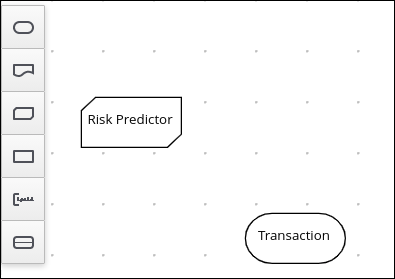1. In the Model window of the DMN editor, drag an input data node to the DMN editor palette.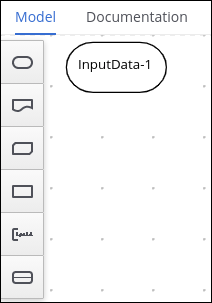2. Rename the node Transaction.

3. Select the node then click the properties pencil icon in the upper-right corner of the window.

4. In the Properties panel, select Information Item → Data type → tTransaction then close the panel.

8. Create the Transaction Dispute Risk decision node and add the Transaction node for data input and the Risk Predictor node for the function: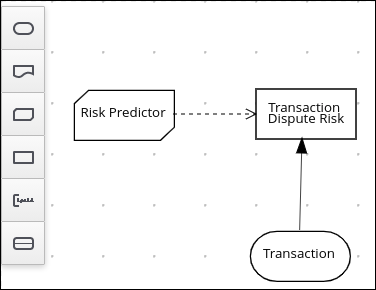1. In the Model window of the DMN editor, drag a decision data node to the DMN editor palette.2. Rename the node Transaction Dispute Risk.

3. Select the Risk Predictor node and drag the arrow from the top right of the node to the Transaction Dispute Risk node.

4. Select the Transaction node and drag the arrow from the bottom right of the node to the Transaction Dispute Risk node.

9. In the Transaction Dispute Risk node, create the Risk predictor invocation function: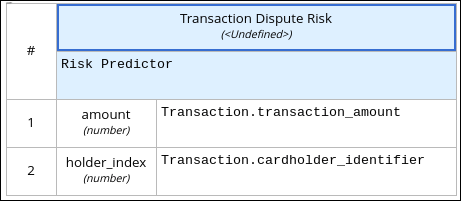1. Select the Transaction Dispute Risk node and click the edit icon on the left side of the node.

2. Click Select expression and select Invocation from the menu.

3. Enter Risk Predictor in the Enter function box.

4. Click P1.

5. In the Edit Parameter dialog, enter `amount` in the Name box, select number from the Data Type menu, and press the Enter key.

6. Click Select expression and select Literal expression from the menu.

7. Enter `Transaction.transaction_amount` in the box next to amount.

8. Right-click on 1 and select Insert below. The Edit Parameter dialog opens.

9. Enter holder_index in the Name box, select number from the Data Type menu, and press the Enter key.

10. Click Select expression on row 2 and select Literal expression from the menu.

11. Enter `Transaction.cardholder_identifier` in the box next to amount.

10. Create the Risk Threshold input data node with the data type number: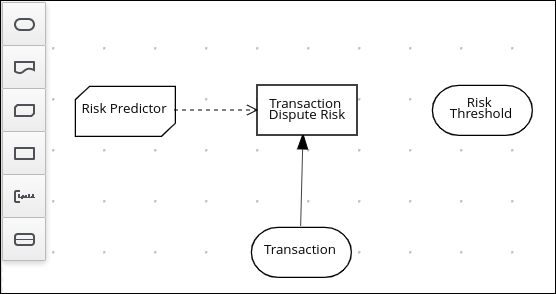1. In the Model window of the DMN editor, drag an input data node to the DMN editor palette.

2. Rename the node Risk Threshold.

3. Select the node then click the properties pencil icon in the upper-right corner of the window.

4. In the Properties panel, select Information Item → Data type → number then close the panel.

11. Create the Can be automatically processed? decision node that takes as inputs the Transaction Dispute Risk and the Risk threshold nodes: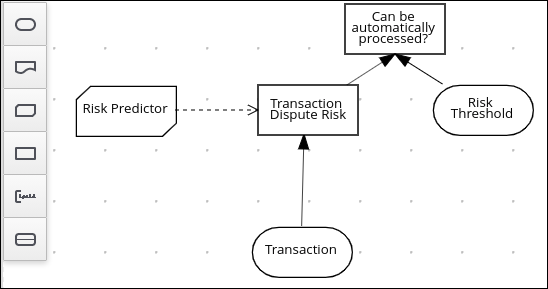1. Drag a decision node to the DMN editor palette and rename it Can be automatically processed?.

2. Select the node, then click the edit icon on the upper-left side of the node.

3. Click Select expression and then select Literal expression from the menu.

4. Enter `Transaction Dispute Risk.predicted_dispute_risk < Risk Threshold` in the box.

5. Select the Transaction Dispute Risk node and drag the arrow in the top left of the node to the Can be automatically processed? node.

6. Select the Risk Threshold node and drag the arrow from the bottom left of the node to the Can be automatically processed? node.

12. Save the model and build the project:

1. In the DMN editor, click Save.

2. If necessary, correct any errors that appear.

4. Click Build. The project should successfully build.

13. Add and run a test scenario: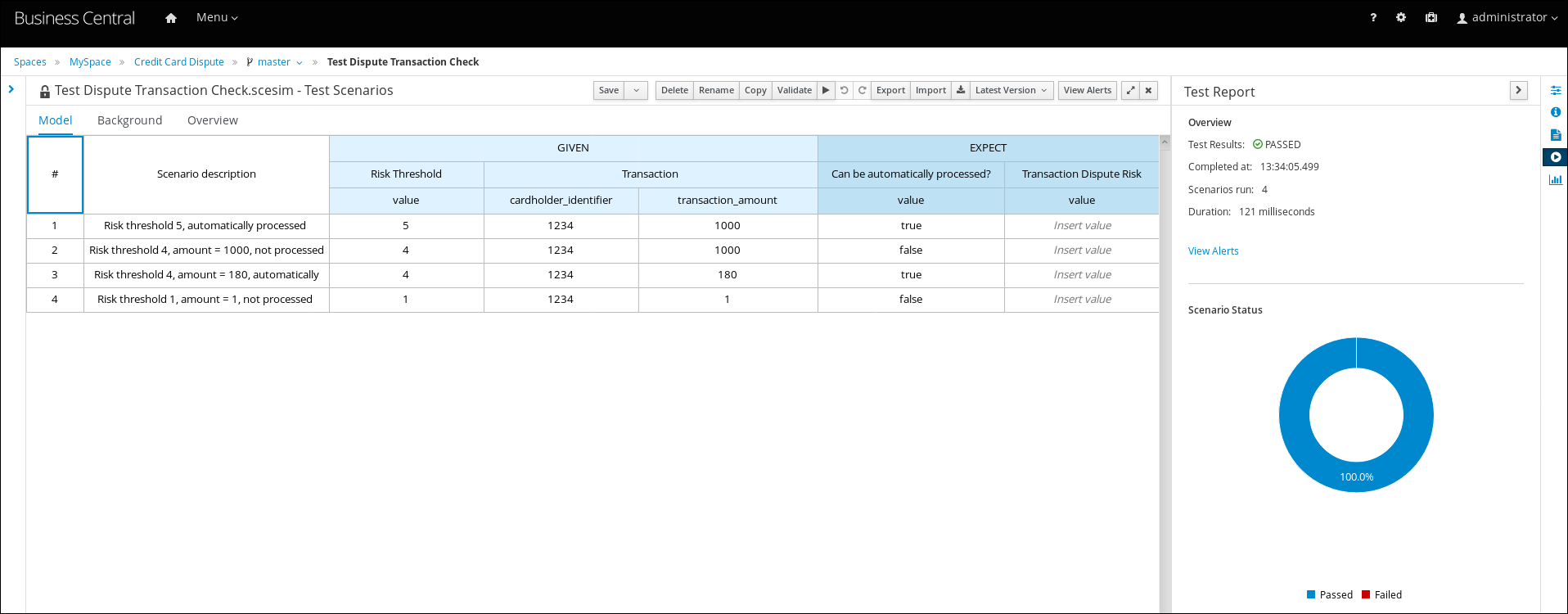1. Create a Test Scenario file.

2. In the Create new Test Scenario dialog, enter the name `Test Dispute Transaction Check`, select com from the Package menu, and select DMN.

3. Select Dispute Transaction Check.dmn from the Choose a DMN asset menu and click OK. The test template builds.

4. Enter the following values and click Save:

 Do not add a value to the Transaction Dispute Risk column. This value is determined by the test scenario.
Table 1. Test scenario parameters
Description Risk Threshold cardholder_identifier transaction_amount Can be automatically processed?

Risk threshold 5, automatically processed

5

1234

1000

true

Risk threshold 4, amount = 1000, not processed

4

1234

1000

false

Risk threshold 4, amount = 180, automatically processed

4

1234

180

true

Risk threshold 1, amount = 1, not processed

1

1234

1

false

5. To run the test, click the Play button in your IDE. The results appear in the Test Report panel on the right of the screen.

### Credit card transaction dispute exercise PMML file

Use the following XML content to create the `dtree_risk_predictor.pmml` file in the Using a PMML model with a DMN model to resolve credit card transaction disputes exercise.

``````<?xml version="1.0" encoding="UTF-8"?>
<PMML xmlns="http://www.dmg.org/PMML-4_2" xmlns:xsi="http://www.w3.org/2001/XMLSchema-instance" version="4.2" xsi:schemaLocation="http://www.dmg.org/PMML-4_2 http://www.dmg.org/v4-2-1/pmml-4-2.xsd">
<Application name="Nyoka" version="4.3.0" />
<Timestamp>2020-10-09 14:27:26.622723</Timestamp>
<DataField name="amount" optype="continuous" dataType="double" />
<DataField name="holder_index" optype="continuous" dataType="double" />
<DataField name="dispute_risk" optype="categorical" dataType="integer">
<Value value="1" />
<Value value="2" />
<Value value="3" />
<Value value="4" />
<Value value="5" />
</DataField>
<TreeModel modelName="DecisionTreeClassifier" functionName="classification" missingValuePenalty="1.0">
<MiningSchema>
<MiningField name="amount" usageType="active" optype="continuous" />
<MiningField name="holder_index" usageType="active" optype="continuous" />
<MiningField name="dispute_risk" usageType="target" optype="categorical" />
</MiningSchema>
<Output>
<OutputField name="probability_1" optype="continuous" dataType="double" feature="probability" value="1" />
<OutputField name="probability_2" optype="continuous" dataType="double" feature="probability" value="2" />
<OutputField name="probability_3" optype="continuous" dataType="double" feature="probability" value="3" />
<OutputField name="probability_4" optype="continuous" dataType="double" feature="probability" value="4" />
<OutputField name="probability_5" optype="continuous" dataType="double" feature="probability" value="5" />
<OutputField name="predicted_dispute_risk" optype="categorical" dataType="integer" feature="predictedValue" />
</Output>
<Node id="0" recordCount="600.0">
<True />
<Node id="1" recordCount="200.0">
<SimplePredicate field="amount" operator="lessOrEqual" value="99.94000244140625" />
<Node id="2" score="2" recordCount="55.0">
<SimplePredicate field="holder_index" operator="lessOrEqual" value="0.5" />
<ScoreDistribution value="1" recordCount="0.0" confidence="0.0" />
<ScoreDistribution value="2" recordCount="55.0" confidence="1.0" />
<ScoreDistribution value="3" recordCount="0.0" confidence="0.0" />
<ScoreDistribution value="4" recordCount="0.0" confidence="0.0" />
<ScoreDistribution value="5" recordCount="0.0" confidence="0.0" />
</Node>
<Node id="3" score="1" recordCount="145.0">
<SimplePredicate field="holder_index" operator="greaterThan" value="0.5" />
<ScoreDistribution value="1" recordCount="145.0" confidence="1.0" />
<ScoreDistribution value="2" recordCount="0.0" confidence="0.0" />
<ScoreDistribution value="3" recordCount="0.0" confidence="0.0" />
<ScoreDistribution value="4" recordCount="0.0" confidence="0.0" />
<ScoreDistribution value="5" recordCount="0.0" confidence="0.0" />
</Node>
</Node>
<Node id="4" recordCount="400.0">
<SimplePredicate field="amount" operator="greaterThan" value="99.94000244140625" />
<Node id="5" recordCount="105.0">
<SimplePredicate field="holder_index" operator="lessOrEqual" value="0.5" />
<Node id="6" score="3" recordCount="54.0">
<SimplePredicate field="amount" operator="lessOrEqual" value="150.4550018310547" />
<ScoreDistribution value="1" recordCount="0.0" confidence="0.0" />
<ScoreDistribution value="2" recordCount="0.0" confidence="0.0" />
<ScoreDistribution value="3" recordCount="54.0" confidence="1.0" />
<ScoreDistribution value="4" recordCount="0.0" confidence="0.0" />
<ScoreDistribution value="5" recordCount="0.0" confidence="0.0" />
</Node>
<Node id="7" recordCount="51.0">
<SimplePredicate field="amount" operator="greaterThan" value="150.4550018310547" />
<Node id="8" recordCount="40.0">
<SimplePredicate field="amount" operator="lessOrEqual" value="200.00499725341797" />
<Node id="9" recordCount="36.0">
<SimplePredicate field="amount" operator="lessOrEqual" value="195.4949951171875" />
<Node id="10" recordCount="2.0">
<SimplePredicate field="amount" operator="lessOrEqual" value="152.2050018310547" />
<Node id="11" score="4" recordCount="1.0">
<SimplePredicate field="amount" operator="lessOrEqual" value="151.31500244140625" />
<ScoreDistribution value="1" recordCount="0.0" confidence="0.0" />
<ScoreDistribution value="2" recordCount="0.0" confidence="0.0" />
<ScoreDistribution value="3" recordCount="0.0" confidence="0.0" />
<ScoreDistribution value="4" recordCount="1.0" confidence="1.0" />
<ScoreDistribution value="5" recordCount="0.0" confidence="0.0" />
</Node>
<Node id="12" score="3" recordCount="1.0">
<SimplePredicate field="amount" operator="greaterThan" value="151.31500244140625" />
<ScoreDistribution value="1" recordCount="0.0" confidence="0.0" />
<ScoreDistribution value="2" recordCount="0.0" confidence="0.0" />
<ScoreDistribution value="3" recordCount="1.0" confidence="1.0" />
<ScoreDistribution value="4" recordCount="0.0" confidence="0.0" />
<ScoreDistribution value="5" recordCount="0.0" confidence="0.0" />
</Node>
</Node>
<Node id="13" recordCount="34.0">
<SimplePredicate field="amount" operator="greaterThan" value="152.2050018310547" />
<Node id="14" recordCount="20.0">
<SimplePredicate field="amount" operator="lessOrEqual" value="176.5050048828125" />
<Node id="15" recordCount="19.0">
<SimplePredicate field="amount" operator="lessOrEqual" value="176.06500244140625" />
<Node id="16" score="4" recordCount="9.0">
<SimplePredicate field="amount" operator="lessOrEqual" value="166.6449966430664" />
<ScoreDistribution value="1" recordCount="0.0" confidence="0.0" />
<ScoreDistribution value="2" recordCount="0.0" confidence="0.0" />
<ScoreDistribution value="3" recordCount="0.0" confidence="0.0" />
<ScoreDistribution value="4" recordCount="9.0" confidence="1.0" />
<ScoreDistribution value="5" recordCount="0.0" confidence="0.0" />
</Node>
<Node id="17" recordCount="10.0">
<SimplePredicate field="amount" operator="greaterThan" value="166.6449966430664" />
<Node id="18" score="3" recordCount="1.0">
<SimplePredicate field="amount" operator="lessOrEqual" value="167.97999572753906" />
<ScoreDistribution value="1" recordCount="0.0" confidence="0.0" />
<ScoreDistribution value="2" recordCount="0.0" confidence="0.0" />
<ScoreDistribution value="3" recordCount="1.0" confidence="1.0" />
<ScoreDistribution value="4" recordCount="0.0" confidence="0.0" />
<ScoreDistribution value="5" recordCount="0.0" confidence="0.0" />
</Node>
<Node id="19" score="4" recordCount="9.0">
<SimplePredicate field="amount" operator="greaterThan" value="167.97999572753906" />
<ScoreDistribution value="1" recordCount="0.0" confidence="0.0" />
<ScoreDistribution value="2" recordCount="0.0" confidence="0.0" />
<ScoreDistribution value="3" recordCount="0.0" confidence="0.0" />
<ScoreDistribution value="4" recordCount="9.0" confidence="1.0" />
<ScoreDistribution value="5" recordCount="0.0" confidence="0.0" />
</Node>
</Node>
</Node>
<Node id="20" score="3" recordCount="1.0">
<SimplePredicate field="amount" operator="greaterThan" value="176.06500244140625" />
<ScoreDistribution value="1" recordCount="0.0" confidence="0.0" />
<ScoreDistribution value="2" recordCount="0.0" confidence="0.0" />
<ScoreDistribution value="3" recordCount="1.0" confidence="1.0" />
<ScoreDistribution value="4" recordCount="0.0" confidence="0.0" />
<ScoreDistribution value="5" recordCount="0.0" confidence="0.0" />
</Node>
</Node>
<Node id="21" score="4" recordCount="14.0">
<SimplePredicate field="amount" operator="greaterThan" value="176.5050048828125" />
<ScoreDistribution value="1" recordCount="0.0" confidence="0.0" />
<ScoreDistribution value="2" recordCount="0.0" confidence="0.0" />
<ScoreDistribution value="3" recordCount="0.0" confidence="0.0" />
<ScoreDistribution value="4" recordCount="14.0" confidence="1.0" />
<ScoreDistribution value="5" recordCount="0.0" confidence="0.0" />
</Node>
</Node>
</Node>
<Node id="22" recordCount="4.0">
<SimplePredicate field="amount" operator="greaterThan" value="195.4949951171875" />
<Node id="23" score="3" recordCount="1.0">
<SimplePredicate field="amount" operator="lessOrEqual" value="195.76499938964844" />
<ScoreDistribution value="1" recordCount="0.0" confidence="0.0" />
<ScoreDistribution value="2" recordCount="0.0" confidence="0.0" />
<ScoreDistribution value="3" recordCount="1.0" confidence="1.0" />
<ScoreDistribution value="4" recordCount="0.0" confidence="0.0" />
<ScoreDistribution value="5" recordCount="0.0" confidence="0.0" />
</Node>
<Node id="24" recordCount="3.0">
<SimplePredicate field="amount" operator="greaterThan" value="195.76499938964844" />
<Node id="25" score="4" recordCount="1.0">
<SimplePredicate field="amount" operator="lessOrEqual" value="196.74500274658203" />
<ScoreDistribution value="1" recordCount="0.0" confidence="0.0" />
<ScoreDistribution value="2" recordCount="0.0" confidence="0.0" />
<ScoreDistribution value="3" recordCount="0.0" confidence="0.0" />
<ScoreDistribution value="4" recordCount="1.0" confidence="1.0" />
<ScoreDistribution value="5" recordCount="0.0" confidence="0.0" />
</Node>
<Node id="26" recordCount="2.0">
<SimplePredicate field="amount" operator="greaterThan" value="196.74500274658203" />
<Node id="27" score="3" recordCount="1.0">
<SimplePredicate field="amount" operator="lessOrEqual" value="197.5800018310547" />
<ScoreDistribution value="1" recordCount="0.0" confidence="0.0" />
<ScoreDistribution value="2" recordCount="0.0" confidence="0.0" />
<ScoreDistribution value="3" recordCount="1.0" confidence="1.0" />
<ScoreDistribution value="4" recordCount="0.0" confidence="0.0" />
<ScoreDistribution value="5" recordCount="0.0" confidence="0.0" />
</Node>
<Node id="28" score="4" recordCount="1.0">
<SimplePredicate field="amount" operator="greaterThan" value="197.5800018310547" />
<ScoreDistribution value="1" recordCount="0.0" confidence="0.0" />
<ScoreDistribution value="2" recordCount="0.0" confidence="0.0" />
<ScoreDistribution value="3" recordCount="0.0" confidence="0.0" />
<ScoreDistribution value="4" recordCount="1.0" confidence="1.0" />
<ScoreDistribution value="5" recordCount="0.0" confidence="0.0" />
</Node>
</Node>
</Node>
</Node>
</Node>
<Node id="29" score="5" recordCount="11.0">
<SimplePredicate field="amount" operator="greaterThan" value="200.00499725341797" />
<ScoreDistribution value="1" recordCount="0.0" confidence="0.0" />
<ScoreDistribution value="2" recordCount="0.0" confidence="0.0" />
<ScoreDistribution value="3" recordCount="0.0" confidence="0.0" />
<ScoreDistribution value="4" recordCount="0.0" confidence="0.0" />
<ScoreDistribution value="5" recordCount="11.0" confidence="1.0" />
</Node>
</Node>
</Node>
<Node id="30" recordCount="295.0">
<SimplePredicate field="holder_index" operator="greaterThan" value="0.5" />
<Node id="31" score="2" recordCount="170.0">
<SimplePredicate field="amount" operator="lessOrEqual" value="150.93499755859375" />
<ScoreDistribution value="1" recordCount="0.0" confidence="0.0" />
<ScoreDistribution value="2" recordCount="170.0" confidence="1.0" />
<ScoreDistribution value="3" recordCount="0.0" confidence="0.0" />
<ScoreDistribution value="4" recordCount="0.0" confidence="0.0" />
<ScoreDistribution value="5" recordCount="0.0" confidence="0.0" />
</Node>
<Node id="32" recordCount="125.0">
<SimplePredicate field="amount" operator="greaterThan" value="150.93499755859375" />
<Node id="33" recordCount="80.0">
<SimplePredicate field="holder_index" operator="lessOrEqual" value="2.5" />
<Node id="34" recordCount="66.0">
<SimplePredicate field="amount" operator="lessOrEqual" value="199.13500213623047" />
<Node id="35" score="3" recordCount="10.0">
<SimplePredicate field="amount" operator="lessOrEqual" value="155.56999969482422" />
<ScoreDistribution value="1" recordCount="0.0" confidence="0.0" />
<ScoreDistribution value="2" recordCount="0.0" confidence="0.0" />
<ScoreDistribution value="3" recordCount="10.0" confidence="1.0" />
<ScoreDistribution value="4" recordCount="0.0" confidence="0.0" />
<ScoreDistribution value="5" recordCount="0.0" confidence="0.0" />
</Node>
<Node id="36" recordCount="56.0">
<SimplePredicate field="amount" operator="greaterThan" value="155.56999969482422" />
<Node id="37" score="2" recordCount="1.0">
<SimplePredicate field="amount" operator="lessOrEqual" value="155.9000015258789" />
<ScoreDistribution value="1" recordCount="0.0" confidence="0.0" />
<ScoreDistribution value="2" recordCount="1.0" confidence="1.0" />
<ScoreDistribution value="3" recordCount="0.0" confidence="0.0" />
<ScoreDistribution value="4" recordCount="0.0" confidence="0.0" />
<ScoreDistribution value="5" recordCount="0.0" confidence="0.0" />
</Node>
<Node id="38" recordCount="55.0">
<SimplePredicate field="amount" operator="greaterThan" value="155.9000015258789" />
<Node id="39" recordCount="31.0">
<SimplePredicate field="amount" operator="lessOrEqual" value="176.3699951171875" />
<Node id="40" recordCount="30.0">
<SimplePredicate field="amount" operator="lessOrEqual" value="175.72000122070312" />
<Node id="41" recordCount="19.0">
<SimplePredicate field="amount" operator="lessOrEqual" value="168.06999969482422" />
<Node id="42" recordCount="6.0">
<SimplePredicate field="amount" operator="lessOrEqual" value="158.125" />
<Node id="43" score="3" recordCount="5.0">
<SimplePredicate field="amount" operator="lessOrEqual" value="157.85499572753906" />
<ScoreDistribution value="1" recordCount="0.0" confidence="0.0" />
<ScoreDistribution value="2" recordCount="0.0" confidence="0.0" />
<ScoreDistribution value="3" recordCount="5.0" confidence="1.0" />
<ScoreDistribution value="4" recordCount="0.0" confidence="0.0" />
<ScoreDistribution value="5" recordCount="0.0" confidence="0.0" />
</Node>
<Node id="44" score="2" recordCount="1.0">
<SimplePredicate field="amount" operator="greaterThan" value="157.85499572753906" />
<ScoreDistribution value="1" recordCount="0.0" confidence="0.0" />
<ScoreDistribution value="2" recordCount="1.0" confidence="1.0" />
<ScoreDistribution value="3" recordCount="0.0" confidence="0.0" />
<ScoreDistribution value="4" recordCount="0.0" confidence="0.0" />
<ScoreDistribution value="5" recordCount="0.0" confidence="0.0" />
</Node>
</Node>
<Node id="45" score="3" recordCount="13.0">
<SimplePredicate field="amount" operator="greaterThan" value="158.125" />
<ScoreDistribution value="1" recordCount="0.0" confidence="0.0" />
<ScoreDistribution value="2" recordCount="0.0" confidence="0.0" />
<ScoreDistribution value="3" recordCount="13.0" confidence="1.0" />
<ScoreDistribution value="4" recordCount="0.0" confidence="0.0" />
<ScoreDistribution value="5" recordCount="0.0" confidence="0.0" />
</Node>
</Node>
<Node id="46" recordCount="11.0">
<SimplePredicate field="amount" operator="greaterThan" value="168.06999969482422" />
<Node id="47" score="2" recordCount="1.0">
<SimplePredicate field="amount" operator="lessOrEqual" value="168.69499969482422" />
<ScoreDistribution value="1" recordCount="0.0" confidence="0.0" />
<ScoreDistribution value="2" recordCount="1.0" confidence="1.0" />
<ScoreDistribution value="3" recordCount="0.0" confidence="0.0" />
<ScoreDistribution value="4" recordCount="0.0" confidence="0.0" />
<ScoreDistribution value="5" recordCount="0.0" confidence="0.0" />
</Node>
<Node id="48" recordCount="10.0">
<SimplePredicate field="amount" operator="greaterThan" value="168.69499969482422" />
<Node id="49" recordCount="4.0">
<SimplePredicate field="holder_index" operator="lessOrEqual" value="1.5" />
<Node id="50" score="2" recordCount="1.0">
<SimplePredicate field="amount" operator="lessOrEqual" value="172.0250015258789" />
<ScoreDistribution value="1" recordCount="0.0" confidence="0.0" />
<ScoreDistribution value="2" recordCount="1.0" confidence="1.0" />
<ScoreDistribution value="3" recordCount="0.0" confidence="0.0" />
<ScoreDistribution value="4" recordCount="0.0" confidence="0.0" />
<ScoreDistribution value="5" recordCount="0.0" confidence="0.0" />
</Node>
<Node id="51" score="3" recordCount="3.0">
<SimplePredicate field="amount" operator="greaterThan" value="172.0250015258789" />
<ScoreDistribution value="1" recordCount="0.0" confidence="0.0" />
<ScoreDistribution value="2" recordCount="0.0" confidence="0.0" />
<ScoreDistribution value="3" recordCount="3.0" confidence="1.0" />
<ScoreDistribution value="4" recordCount="0.0" confidence="0.0" />
<ScoreDistribution value="5" recordCount="0.0" confidence="0.0" />
</Node>
</Node>
<Node id="52" score="3" recordCount="6.0">
<SimplePredicate field="holder_index" operator="greaterThan" value="1.5" />
<ScoreDistribution value="1" recordCount="0.0" confidence="0.0" />
<ScoreDistribution value="2" recordCount="0.0" confidence="0.0" />
<ScoreDistribution value="3" recordCount="6.0" confidence="1.0" />
<ScoreDistribution value="4" recordCount="0.0" confidence="0.0" />
<ScoreDistribution value="5" recordCount="0.0" confidence="0.0" />
</Node>
</Node>
</Node>
</Node>
<Node id="53" score="2" recordCount="1.0">
<SimplePredicate field="amount" operator="greaterThan" value="175.72000122070312" />
<ScoreDistribution value="1" recordCount="0.0" confidence="0.0" />
<ScoreDistribution value="2" recordCount="1.0" confidence="1.0" />
<ScoreDistribution value="3" recordCount="0.0" confidence="0.0" />
<ScoreDistribution value="4" recordCount="0.0" confidence="0.0" />
<ScoreDistribution value="5" recordCount="0.0" confidence="0.0" />
</Node>
</Node>
<Node id="54" recordCount="24.0">
<SimplePredicate field="amount" operator="greaterThan" value="176.3699951171875" />
<Node id="55" score="3" recordCount="16.0">
<SimplePredicate field="amount" operator="lessOrEqual" value="192.0999984741211" />
<ScoreDistribution value="1" recordCount="0.0" confidence="0.0" />
<ScoreDistribution value="2" recordCount="0.0" confidence="0.0" />
<ScoreDistribution value="3" recordCount="16.0" confidence="1.0" />
<ScoreDistribution value="4" recordCount="0.0" confidence="0.0" />
<ScoreDistribution value="5" recordCount="0.0" confidence="0.0" />
</Node>
<Node id="56" recordCount="8.0">
<SimplePredicate field="amount" operator="greaterThan" value="192.0999984741211" />
<Node id="57" score="2" recordCount="1.0">
<SimplePredicate field="amount" operator="lessOrEqual" value="192.75499725341797" />
<ScoreDistribution value="1" recordCount="0.0" confidence="0.0" />
<ScoreDistribution value="2" recordCount="1.0" confidence="1.0" />
<ScoreDistribution value="3" recordCount="0.0" confidence="0.0" />
<ScoreDistribution value="4" recordCount="0.0" confidence="0.0" />
<ScoreDistribution value="5" recordCount="0.0" confidence="0.0" />
</Node>
<Node id="58" score="3" recordCount="7.0">
<SimplePredicate field="amount" operator="greaterThan" value="192.75499725341797" />
<ScoreDistribution value="1" recordCount="0.0" confidence="0.0" />
<ScoreDistribution value="2" recordCount="0.0" confidence="0.0" />
<ScoreDistribution value="3" recordCount="7.0" confidence="1.0" />
<ScoreDistribution value="4" recordCount="0.0" confidence="0.0" />
<ScoreDistribution value="5" recordCount="0.0" confidence="0.0" />
</Node>
</Node>
</Node>
</Node>
</Node>
</Node>
<Node id="59" recordCount="14.0">
<SimplePredicate field="amount" operator="greaterThan" value="199.13500213623047" />
<Node id="60" score="5" recordCount="10.0">
<SimplePredicate field="holder_index" operator="lessOrEqual" value="1.5" />
<ScoreDistribution value="1" recordCount="0.0" confidence="0.0" />
<ScoreDistribution value="2" recordCount="0.0" confidence="0.0" />
<ScoreDistribution value="3" recordCount="0.0" confidence="0.0" />
<ScoreDistribution value="4" recordCount="0.0" confidence="0.0" />
<ScoreDistribution value="5" recordCount="10.0" confidence="1.0" />
</Node>
<Node id="61" score="4" recordCount="4.0">
<SimplePredicate field="holder_index" operator="greaterThan" value="1.5" />
<ScoreDistribution value="1" recordCount="0.0" confidence="0.0" />
<ScoreDistribution value="2" recordCount="0.0" confidence="0.0" />
<ScoreDistribution value="3" recordCount="0.0" confidence="0.0" />
<ScoreDistribution value="4" recordCount="4.0" confidence="1.0" />
<ScoreDistribution value="5" recordCount="0.0" confidence="0.0" />
</Node>
</Node>
</Node>
<Node id="62" recordCount="45.0">
<SimplePredicate field="holder_index" operator="greaterThan" value="2.5" />
<Node id="63" score="2" recordCount="37.0">
<SimplePredicate field="amount" operator="lessOrEqual" value="199.13999938964844" />
<ScoreDistribution value="1" recordCount="0.0" confidence="0.0" />
<ScoreDistribution value="2" recordCount="37.0" confidence="1.0" />
<ScoreDistribution value="3" recordCount="0.0" confidence="0.0" />
<ScoreDistribution value="4" recordCount="0.0" confidence="0.0" />
<ScoreDistribution value="5" recordCount="0.0" confidence="0.0" />
</Node>
<Node id="64" score="4" recordCount="8.0">
<SimplePredicate field="amount" operator="greaterThan" value="199.13999938964844" />
<ScoreDistribution value="1" recordCount="0.0" confidence="0.0" />
<ScoreDistribution value="2" recordCount="0.0" confidence="0.0" />
<ScoreDistribution value="3" recordCount="0.0" confidence="0.0" />
<ScoreDistribution value="4" recordCount="8.0" confidence="1.0" />
<ScoreDistribution value="5" recordCount="0.0" confidence="0.0" />
</Node>
</Node>
</Node>
</Node>
</Node>
</Node>
</TreeModel>
</PMML>``````